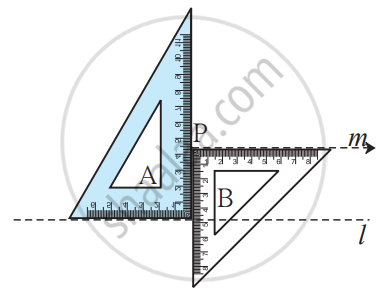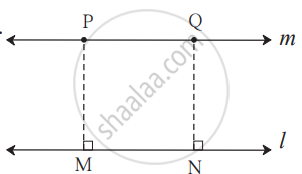# To Draw a Line Parallel to the Given Line Through a Point Outside the Given Line Using Set-square.

## Notes

### To draw a line parallel to the given line through a point outside the given line using set - square:

• #### Method I: Steps of the construction1. Draw line l.
2. Take a point P outside the line l.
3. As shown in the figure, place two sets-squares touching each other. Hold set - squares A and B. One edge of set-square A is close to point P. Draw a line along the edge of B.
4. Name the line as m.
5. Line m is parallel to line l.
• #### Method II: Steps of construction1. Draw line l. Take a point P outside the line.
2. Draw a seg PM ⊥ line l.
3.  Take another point N on line l.
4. Draw seg NQ ⊥ line l. such that l("NQ") = l("MP").
5. The line m passing through points P and Q is parallel to line l.
If you would like to contribute notes or other learning material, please submit them using the button below.

### Shaalaa.com

To Draw a Line Parallel to the Given Line Through a Point Outside the Given Line Using Set-square. [00:03:04]
S
0%